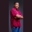Related Tags

c#
communitycreator

# How to calculate the volume of a cone in C#Theodore Kelechukwu Onyejiaku

## Overview

The volume of a cone is the total amount of space that the cone occupies in a three-dimensional plane. The product of Pi (π), the cone's height, the square of its radius, and 1/3 will give us the volume of a cone.

We can easily calculate a cone's volume in C#.

### Syntax

(1.0 / 3) * Math.PI * r * r * h;
Syntax for getting the volume of a cone in C#

### Variables

• Math.PI: This is the mathematical constant Pi.
• r: This is the radius of the cone.
• h: This is the height of the cone.

### Return value

The value returned is a double value, representing the volume of the specified cone.

### Example

using System;
class ConeVolume
{
// Create a function to get the areas
static void GetVolume(double h, double r){
double volume = (1.0 / 3) * Math.PI * r * r * h;
Console.WriteLine("The volume of the cone with height " + h + " and radius " + r + " is " + volume);
}

// The main method
static void Main()
{
// Create the radii of some cones
double r1 = 10;
double r2 = 1.5;
double r3 = 4.5;

// Create the heights of the cones
double h1 = 2.2;
double h2 = 5;
double h3 = 20.5;

// Get the volumes of the cones
GetVolume(h1, r1);
GetVolume(h2, r2);
GetVolume(h3, r3);
}
}
Getting the volume of a cone in C#

### Explanation

• Line 5: We create a method called GetVolume(). It takes two parameters: height and radius. This method calculates the area of the cone and shows us the result on the console.
• Lines 14–16: Inside the Main() function, we create the radii of the cones whose volume we want to get.
• Lines 19–21: We create the heights of the cones.
• Lines 24–26: We invoke the GetVolume() method, and pass the heights and radii we created. Then, this method reveals the volume on the console.

RELATED TAGS

c#
communitycreator

CONTRIBUTORTheodore Kelechukwu Onyejiaku
RELATED COURSES

View all Courses

Keep Exploring

Learn in-demand tech skills in half the time